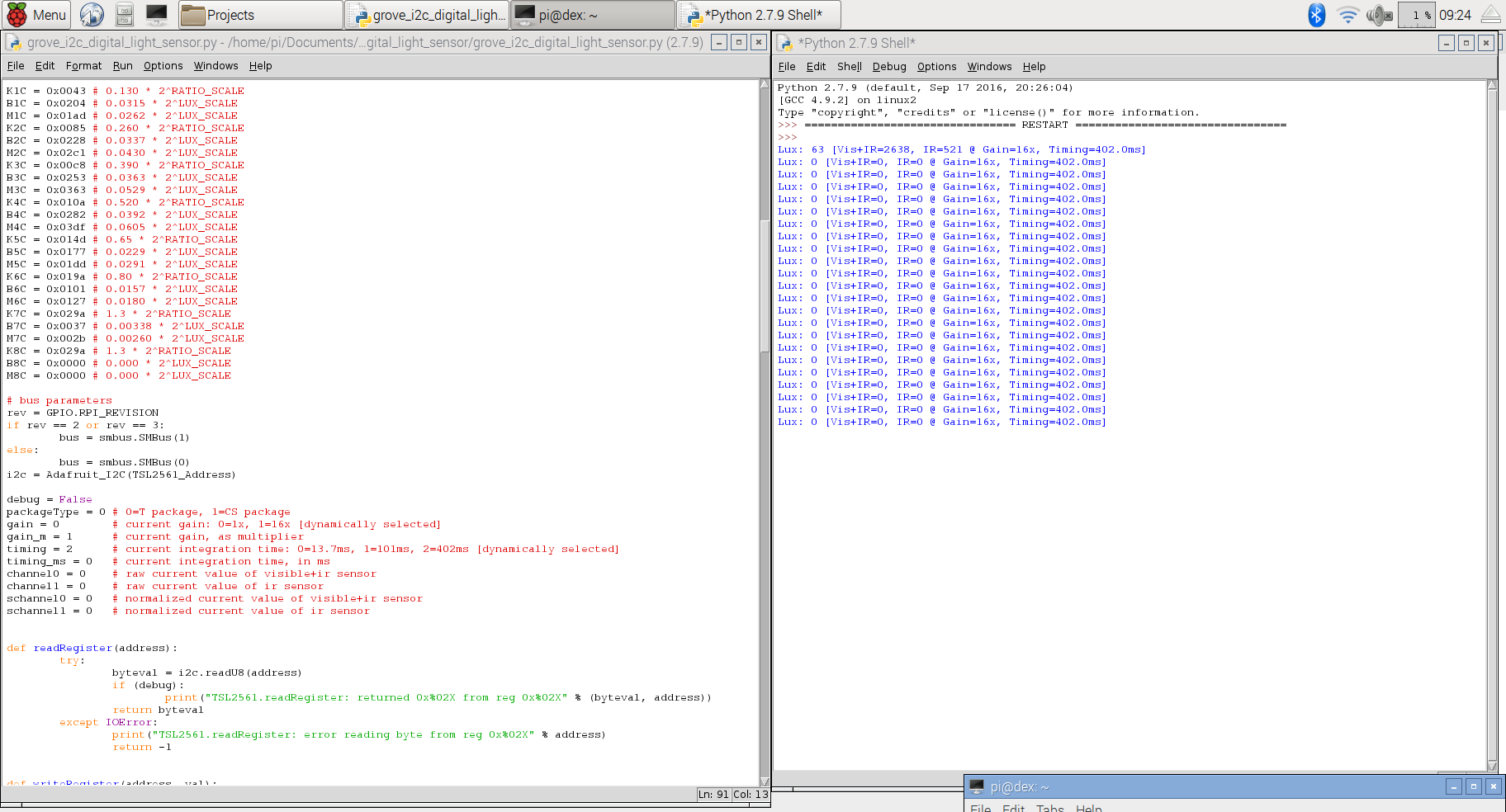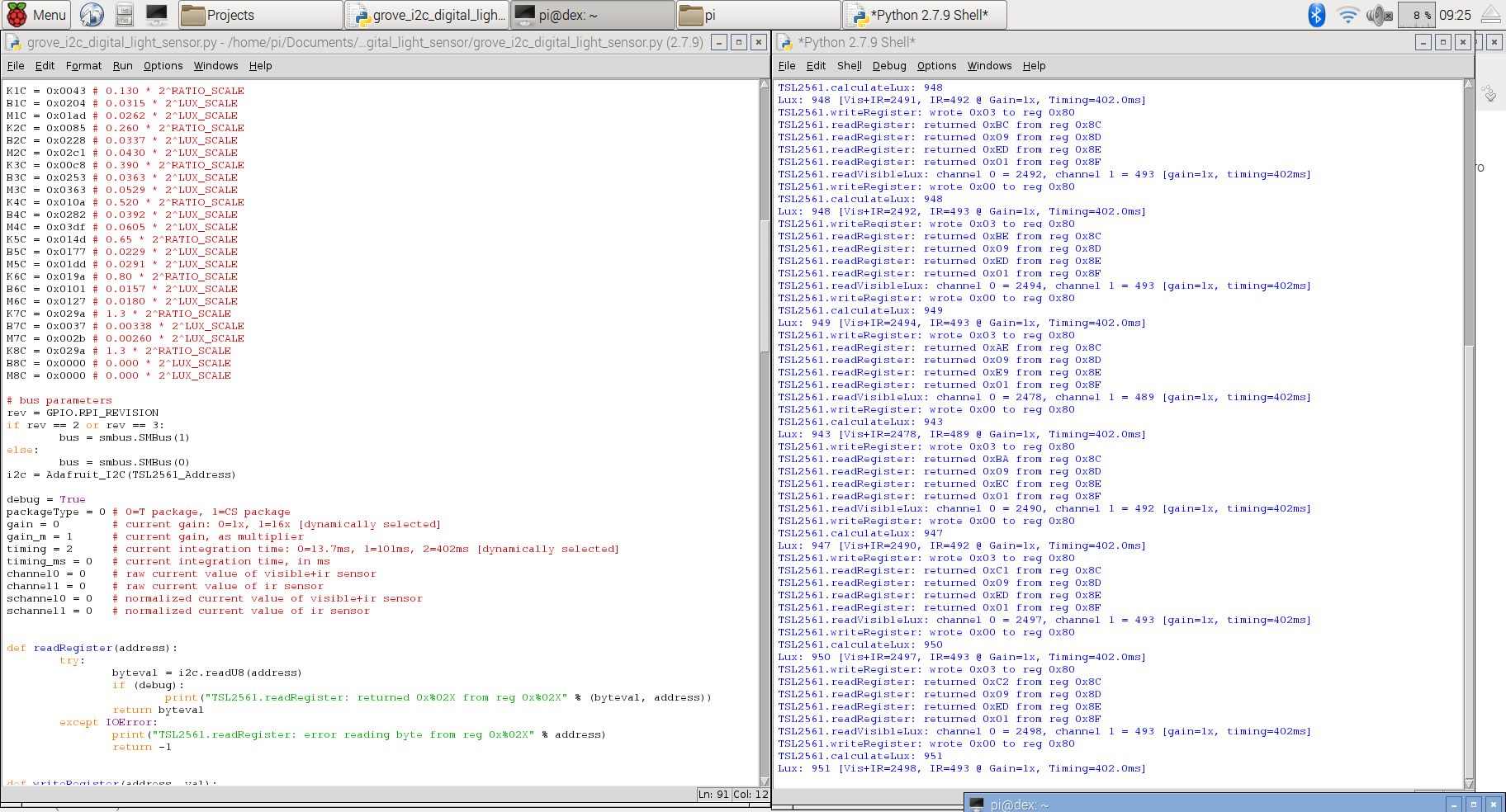# [SOLVED] Grove I2C Digital Light Sensor problem

Hey guys,
Im trying to use the Digital Light Sensor with the grove pi. However when I try and use it won’t work. I tried running the grove_i2c_digital_light_sensor.py program but it gives one reading of about 54lux then all other readings are 0 for everything. The strange thing is that when I turn the debug on it seems to suddenly work, giving readings of about 800lux, so I don’t think the sensor is broke or anything. I’m very confused and was wondering if anyone could help.

Hi @cfinnegan12,

Can you show us some screenshots of what you get?
Say, screenshots before and after setting `debug` variable to `True`.

Also, I suppose you’re using this `Python` script:

Thank you!

Here are screenshots of the debug on true and false. And yes Im using that Python script. ThanksHi @cfinnegan12,

I think we may have a timing issue.

When `debug = True` is set, the `prints` we have take some time to get executed.
This may be enough to get the `I2C` device ready for communication again.

But when we have `debug = False`, the program is running a little faster, which may be too much for the `I2C` device to “handle”.

I think we need to add some delays before any print statements (which should be out of the `if debug:` conditionals) and see if it works.
I’ve set a `global` variable called `cooldown_time` to `0.005` seconds.

Please try it out and see if the program starts working again.
If not, please increase the `cooldown_time` to `0.010` seconds.

Here’s the modified source code you need to run:

``````#!/usr/bin/python
# TSL2561 I2C Light-To-Digital converter library for the Raspberry Pi.
#
# This library is based on the work by Cedric Maion https://github.com/cmaion/TSL2561
#

import time
import smbus
import RPi.GPIO as GPIO
from smbus import SMBus

TSL2561_Control = 0x80
TSL2561_Timing = 0x81
TSL2561_Interrupt = 0x86
TSL2561_Channel0L = 0x8C
TSL2561_Channel0H = 0x8D
TSL2561_Channel1L = 0x8E
TSL2561_Channel1H = 0x8F

LUX_SCALE = 14 # scale by 2^14
RATIO_SCALE = 9 # scale ratio by 2^9
CH_SCALE = 10 # scale channel values by 2^10
CHSCALE_TINT0 = 0x7517 # 322/11 * 2^CH_SCALE
CHSCALE_TINT1 = 0x0fe7 # 322/81 * 2^CH_SCALE

K1T = 0x0040 # 0.125 * 2^RATIO_SCALE
B1T = 0x01f2 # 0.0304 * 2^LUX_SCALE
M1T = 0x01be # 0.0272 * 2^LUX_SCALE
K2T = 0x0080 # 0.250 * 2^RATIO_SCA
B2T = 0x0214 # 0.0325 * 2^LUX_SCALE
M2T = 0x02d1 # 0.0440 * 2^LUX_SCALE
K3T = 0x00c0 # 0.375 * 2^RATIO_SCALE
B3T = 0x023f # 0.0351 * 2^LUX_SCALE
M3T = 0x037b # 0.0544 * 2^LUX_SCALE
K4T = 0x0100 # 0.50 * 2^RATIO_SCALE
B4T = 0x0270 # 0.0381 * 2^LUX_SCALE
M4T = 0x03fe # 0.0624 * 2^LUX_SCALE
K5T = 0x0138 # 0.61 * 2^RATIO_SCALE
B5T = 0x016f # 0.0224 * 2^LUX_SCALE
M5T = 0x01fc # 0.0310 * 2^LUX_SCALE
K6T = 0x019a # 0.80 * 2^RATIO_SCALE
B6T = 0x00d2 # 0.0128 * 2^LUX_SCALE
M6T = 0x00fb # 0.0153 * 2^LUX_SCALE
K7T = 0x029a # 1.3 * 2^RATIO_SCALE
B7T = 0x0018 # 0.00146 * 2^LUX_SCALE
M7T = 0x0012 # 0.00112 * 2^LUX_SCALE
K8T = 0x029a # 1.3 * 2^RATIO_SCALE
B8T = 0x0000 # 0.000 * 2^LUX_SCALE
M8T = 0x0000 # 0.000 * 2^LUX_SCALE

K1C = 0x0043 # 0.130 * 2^RATIO_SCALE
B1C = 0x0204 # 0.0315 * 2^LUX_SCALE
M1C = 0x01ad # 0.0262 * 2^LUX_SCALE
K2C = 0x0085 # 0.260 * 2^RATIO_SCALE
B2C = 0x0228 # 0.0337 * 2^LUX_SCALE
M2C = 0x02c1 # 0.0430 * 2^LUX_SCALE
K3C = 0x00c8 # 0.390 * 2^RATIO_SCALE
B3C = 0x0253 # 0.0363 * 2^LUX_SCALE
M3C = 0x0363 # 0.0529 * 2^LUX_SCALE
K4C = 0x010a # 0.520 * 2^RATIO_SCALE
B4C = 0x0282 # 0.0392 * 2^LUX_SCALE
M4C = 0x03df # 0.0605 * 2^LUX_SCALE
K5C = 0x014d # 0.65 * 2^RATIO_SCALE
B5C = 0x0177 # 0.0229 * 2^LUX_SCALE
M5C = 0x01dd # 0.0291 * 2^LUX_SCALE
K6C = 0x019a # 0.80 * 2^RATIO_SCALE
B6C = 0x0101 # 0.0157 * 2^LUX_SCALE
M6C = 0x0127 # 0.0180 * 2^LUX_SCALE
K7C = 0x029a # 1.3 * 2^RATIO_SCALE
B7C = 0x0037 # 0.00338 * 2^LUX_SCALE
M7C = 0x002b # 0.00260 * 2^LUX_SCALE
K8C = 0x029a # 1.3 * 2^RATIO_SCALE
B8C = 0x0000 # 0.000 * 2^LUX_SCALE
M8C = 0x0000 # 0.000 * 2^LUX_SCALE

# bus parameters
rev = GPIO.RPI_REVISION
if rev == 2 or rev == 3:
bus = smbus.SMBus(1)
else:
bus = smbus.SMBus(0)

debug = False
cooldown_time = 0.005
packageType = 0 # 0=T package, 1=CS package
gain = 0        # current gain: 0=1x, 1=16x [dynamically selected]
gain_m = 1      # current gain, as multiplier
timing = 2      # current integration time: 0=13.7ms, 1=101ms, 2=402ms [dynamically selected]
timing_ms = 0   # current integration time, in ms
channel0 = 0    # raw current value of visible+ir sensor
channel1 = 0    # raw current value of ir sensor
schannel0 = 0   # normalized current value of visible+ir sensor
schannel1 = 0   # normalized current value of ir sensor

try:

sleep(cooldown_time)
if (debug):
return byteval
except IOError:
return -1

try:

sleep(cooldown_time)
if (debug):
print("TSL2561.writeRegister: wrote 0x%02X to reg 0x%02X" % (val, address))
except IOError:

sleep(cooldown_time)
print("TSL2561.writeRegister: error writing byte to reg 0x%02X" % address)
return -1

def powerUp():
writeRegister(TSL2561_Control, 0x03)

def powerDown():
writeRegister(TSL2561_Control, 0x00)

def setTintAndGain():
global gain_m, timing_ms

if gain == 0:
gain_m = 1
else:
gain_m = 16

if timing == 0:
timing_ms = 13.7
elif timing == 1:
timing_ms = 101
else:
timing_ms = 402
writeRegister(TSL2561_Timing, timing | gain << 4)

time.sleep(float(timing_ms + 1) / 1000)

global channel0, channel1
channel0 = (ch0_high<<8) | ch0_low
channel1 = (ch1_high<<8) | ch1_low

sleep(cooldown_time)
if debug:
print("TSL2561.readVisibleLux: channel 0 = %i, channel 1 = %i [gain=%ix, timing=%ims]" % (channel0, channel1, gain_m, timing_ms))

global timing, gain

powerUp()

if channel0 < 500 and timing == 0:
timing = 1
sleep(cooldown_time)
if debug:
print("TSL2561.readVisibleLux: too dark. Increasing integration time from 13.7ms to 101ms")
setTintAndGain()

if channel0 < 500 and timing == 1:
timing = 2
sleep(cooldown_time)
if debug:
print("TSL2561.readVisibleLux: too dark. Increasing integration time from 101ms to 402ms")
setTintAndGain()

if channel0 < 500 and timing == 2 and gain == 0:
gain = 1
sleep(cooldown_time)
if debug:
print("TSL2561.readVisibleLux: too dark. Setting high gain")
setTintAndGain()

if (channel0 > 20000 or channel1 > 20000) and timing == 2 and gain == 1:
gain = 0
sleep(cooldown_time)
if debug:
print("TSL2561.readVisibleLux: enough light. Setting low gain")
setTintAndGain()

if (channel0 > 20000 or channel1 > 20000) and timing == 2:
timing = 1
sleep(cooldown_time)
if debug:
print("TSL2561.readVisibleLux: enough light. Reducing integration time from 402ms to 101ms")
setTintAndGain()

if (channel0 > 10000 or channel1 > 10000) and timing == 1:
timing = 0
sleep(cooldown_time)
if debug:
print("TSL2561.readVisibleLux: enough light. Reducing integration time from 101ms to 13.7ms")
setTintAndGain()

powerDown()

if (timing == 0 and (channel0 > 5000 or channel1 > 5000)) or (timing == 1 and (channel0 > 37000 or channel1 > 37000)) or (timing == 2 and (channel0 > 65000 or channel1 > 65000)):
# overflow
return -1

return calculateLux(channel0, channel1)

def calculateLux(ch0, ch1):
chScale = 0
if timing == 0:   # 13.7 msec
chScale = CHSCALE_TINT0
elif timing == 1: # 101 msec
chScale = CHSCALE_TINT1;
else:           # assume no scaling
chScale = (1 << CH_SCALE)

if gain == 0:
chScale = chScale << 4 # scale 1X to 16X

# scale the channel values
global schannel0, schannel1
schannel0 = (ch0 * chScale) >> CH_SCALE
schannel1 = (ch1 * chScale) >> CH_SCALE

ratio = 0
if schannel0 != 0:
ratio = (schannel1 << (RATIO_SCALE+1)) / schannel0
ratio = (ratio + 1) >> 1

if packageType == 0: # T package
if ((ratio >= 0) and (ratio <= K1T)):
b=B1T; m=M1T;
elif (ratio <= K2T):
b=B2T; m=M2T;
elif (ratio <= K3T):
b=B3T; m=M3T;
elif (ratio <= K4T):
b=B4T; m=M4T;
elif (ratio <= K5T):
b=B5T; m=M5T;
elif (ratio <= K6T):
b=B6T; m=M6T;
elif (ratio <= K7T):
b=B7T; m=M7T;
elif (ratio > K8T):
b=B8T; m=M8T;
elif packageType == 1: # CS package
if ((ratio >= 0) and (ratio <= K1C)):
b=B1C; m=M1C;
elif (ratio <= K2C):
b=B2C; m=M2C;
elif (ratio <= K3C):
b=B3C; m=M3C;
elif (ratio <= K4C):
b=B4C; m=M4C;
elif (ratio <= K5C):
b=B5C; m=M5C;
elif (ratio <= K6C):
b=B6C; m=M6C;
elif (ratio <= K7C):
b=B7C; m=M7C;

temp = ((schannel0*b)-(schannel1*m))
if temp < 0:
temp = 0;
temp += (1<<(LUX_SCALE-1))
# strip off fractional portion
lux = temp>>LUX_SCALE
sleep(cooldown_time)
if debug:
print("TSL2561.calculateLux: %i" % lux)

return lux

def init():
powerUp()
setTintAndGain()
writeRegister(TSL2561_Interrupt, 0x00)
powerDown()

def main():
init()
while (True):
print("Lux: %i [Vis+IR=%i, IR=%i @ Gain=%ix, Timing=%.1fms]" % (readVisibleLux(), channel0, channel1, gain_m, timing_ms))
time.sleep(1)

if __name__ == "__main__":
main()
``````

After you test the `Python` script, please tell us if it solves your issue.
We may need to correct this issue in our repository too.

Thank you!

Your solution worked! You were spot on, its working fine now after introducing the delay. Thank you very much!

Hi @cfinnegan12,

I’m glad to hear the solution worked for you.
I’m in the process of making a `Pull Request` to our main `repo` with these changes.

In `1-2` days you’ll be able to update your `GoPiGo` repo with these new changes.

Can we close the thread now?

Thank you!

A post was split to a new topic: Grove I2C Digital Light Sensor throwing high values